# NanoBlog

## Skywater 130nm Advanced Process Design Workshop

morreale Thursday 11 of August, 2022efabless sponsored a workshop on Advanced Physical Design using the openLANE open source toolchain, and the Skywater 130 nm Physical Design Kit (PDK). Google and Skywater have been working to make custom semiconductor design and processing open source. The course was produced and taught by VLSI System Design (VSD) - Intelligent Assessment Technology (IAT).

The workshop was held August 2 to 6 and each day begins with an Q&A session at 10:30 AM ET over Zoom. The course lectures were recorded and made available on the VSD learning platform. The platform also provided a compute instance containing all the tools and PDK library all setup to use.

The platform was very responsive for both the lecture recordings and running the labs to learn the design toolchain. The workshop also provided a Slack workspace to interact with other students and get questions answered. The instructors were very good about responding to questions to help solve problems with simulations. The subjects covered each day are shown below. Each module also had exams.

• Day 1 - Inception of open-source EDA, OpenLANE and Sky130 PDK
• Day 2 - Good floorplan vs bad floorplan and introduction to library cells
• Day 3 - Design library cell using Magic Layout and ngspice characterization
• Day 4 - Pre-layout timing analysis and importance of good clock tree
• Day 5 - Final steps for RTL2GDS using tritonRoute and openSTA

The first three days introduce you to the openLANE toolchain and the PDK. On the last two days, we replaced a standard cell inverter with a custom cell in the picorv32a design to demonstrate how to add a custom cell to an existing design. The picorv32a is a Size-Optimized RISC-V CPU core that executes the RISC-V RV32IMC Instruction Set. The sections below describe show my results produced from the workshop labs. The workshop is intense and required around 10 to 12 hrs/day to work through the lectures, labs and exams as this was my first introduction to an ASIC design toolchain.

There wasn't enough time to finish the timing optimization. Fortunately, the tools can be downloaded and installed on a local computer and completed offline. Designs can be submitted to the Google/Skywater Multi-Project Wafer (MPW) shuttle. The submission deadline is Sept 12, 2022. To learn more, please see my Skywater 130n Advanced Process Design Workshop report on GitHub. My certificate of completion is shown below.## Emerging Power Management Solutions Course

morreale Tuesday 31 of May, 2022

The Emerging Power Management Systems short course was held from May 3 to May26, 2022 virtually through the University of Limerick. The short course consists of eight 2 hour lectures followed by a question and answer session. The course is taught by Aleksandar Prodic from the University of Toronto.

The course was fascinating. The professor described the Small Ripple Approximation (SRA), the Inductor Volt-Second Balance, and Capacitor-Charge Balance methods of analyzing Switch Mode Power Supplies (SMPSs). The professor used the approximation on boost and buck converters to create an equivalent circuits that replaced the switching elements with a transformer with a winding ratio related to the duty cycle of the controller plus some voltage and current sources. Then the model can be simulated in SPICE using AC analysis without complex switching signals to worry about.

## Course Program

The course program outline is shown below:

• Lecture 1 - Power Management Systems
• Topologies, SMPS, linear regulators, converters, SC circuits, design trade-offs, converter modeling.
• Lecture 2 - Controller Design – Analog vs. Digital
• Analog controller design, motivation & challenges, high-frequency digital controllers.|
• Lecture 3 - High-Frequency Digital Controllers
• Practical implementation, limit cycling & resolution, design methods, compensator design, on-chip implementation.
• Lecture 4 - High-Performance Controllers
• Advanced digital & mixed-signal controllers, time-optimal & minimum deviation control, on-line efficiency optimization, load-interactive features.
• Lecture 5 - Emerging Converter Topologies
• Limitations of conventional solutions, reduced voltage swing principle, multi-level (ML) converter topologies, principles of operation & analysis.
• Lecture 6 - Control of Multi-Level Controllers – Part I
• Design & control challenges, flying capacitor (FC) voltage control, start-up issues.
• Lecture 7 - Control of Multi-Level Controllers – Part II
• Practical mixed-signal controllers for ML-FC SMPS, advanced features, design example.
• Lecture 8 - Advanced Power Management Architectures
• Hybrid architectures, design example, on-chip implementation of PM system for mobile applications.

## Text book

One of the text books recommended for the course was the Fundamentals of Power Electronics by R. W. Erickson and D. Maksimovic.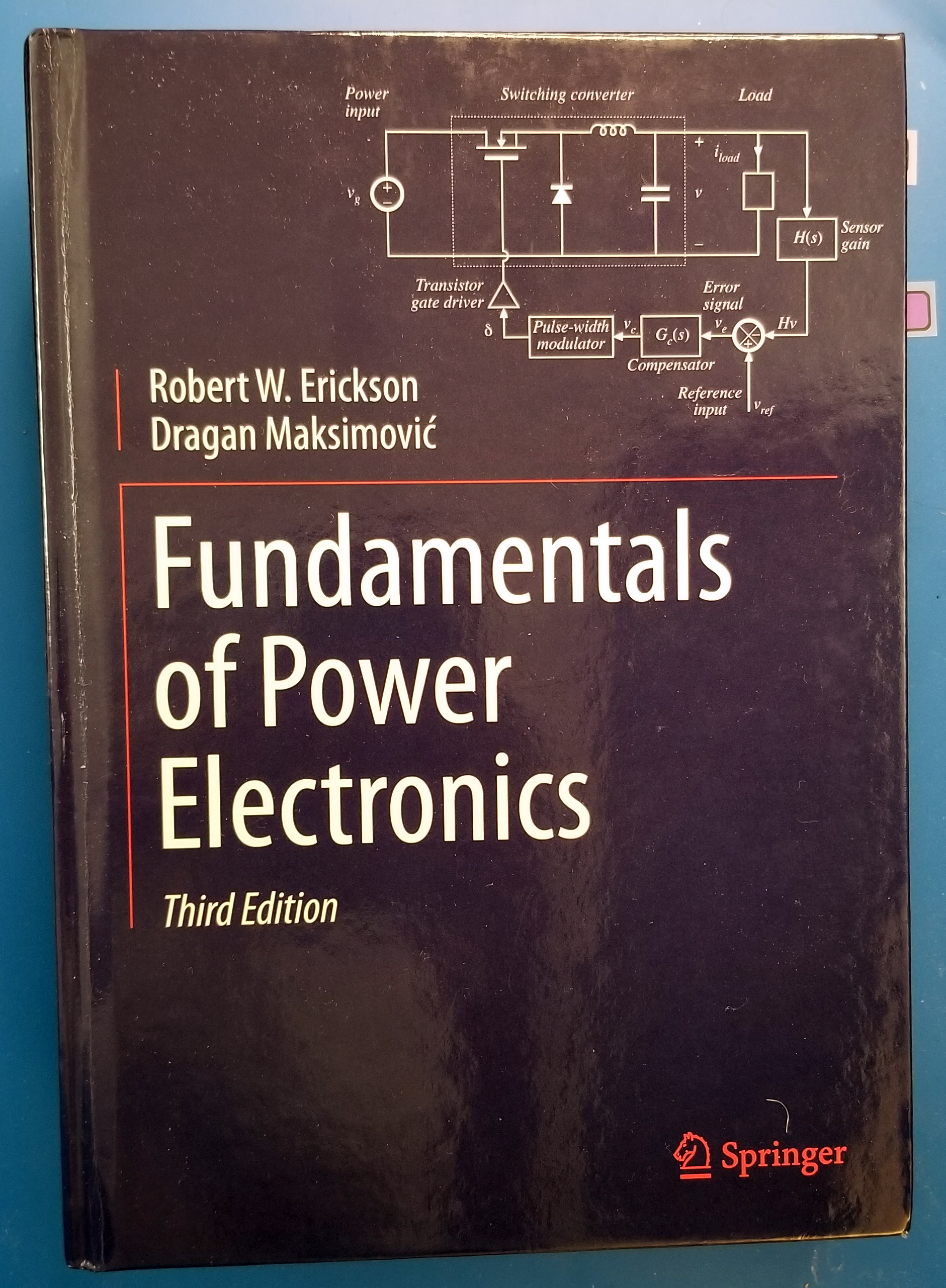## Simulators

We used the Typhoon-HIL Control Center power simulator with the Sandia National Laboratories Xyce SPICE simulator for our homework assignments. The Xyce simulator was selected because it is well suited for simulating switching power converters.

## Acknowledgements

I enjoyed this course. I appreciate Professor Prodic for teaching the course, and Hooman Reyhani for his efforts organizing the course.

## Certificate of Completion## References

1. R. W. Erickson and D. Maksimovic, Fundamentals of Power Electronics, 3rd edition, ISBN-10: 3030438791, Aug. 2020, or 2nd edition Springer Media Inc., 2001.
2. ISTE - Wiley, Power Systems-On-Chip: Practical Aspects of Design, edited by Bruno Allard, ISBN: 978-1-786-30081-2, 2016.
3. A Prodic, D Maksimovic, RW Erickson, Design and implementation of a digital PWM controller for a high-frequency switching DC-DC power converter, in Proc. IEEE IECON’01, 2001.
4. A Prodic, D Maksimovic, Design of a digital PID regulator based on look-up tables for control of high-frequency DC-DC converters, in Proc IEEE COMPEL 2002.
5. AV Peterchev, SR Sanders, Quantization resolution and limit cycling in digitally controlled PWM converters, IEEE Trans. on Power Electronics, 2003.
6. H Peng, A Prodic, E Alarcón, D Maksimovic, Modeling of quantization effects in digitally controlled dc–dc converters, IEEE Trans. on power electronics, 2007.
7. Z Lukic, N Rahman, A Prodic, Multibit $\Sigma-\Delta$ PWM Digital Controller IC for DC–DC Converters Operating at Switching Frequencies Beyond 10 MHz, IEEE Trans. on Power Electronics 22 (5), 2007.
8. T.A. Meynard, H. Foch, Multi-level conversion: high voltage choppers and voltage-source inverters, Power Electronics Specialists Conference, 1992. PESC '92 Record.
9. T.A Meynard, H. Foch, Multilevel converters and derived topologies for high power conversion, Industrial Electronics, Control, and Instrumentation, 1995., Proceedings of the 1995 IEEE IECON 21st International Conference.
10. T.A. Meynard, H. Foch, P. Thomas, J. Courault, R. Jakob and M. Nahrstaedt, Multicell converters: basic concepts and industry applications, Industrial Electronics, IEEE Trans. on, 2002.
11. P. S. Shenoy, M. Amaro, J. Morroni and D. Freeman, Comparison of a Buck Converter and a Series Capacitor Buck Converter for High-Frequency, High-Conversion-Ratio Voltage Regulators, IEEE Trans. on Power Electronics, 2016.
12. K. Nishijima, K. Harada, T. Nakano, T. Nabeshima and T. Sato, Analysis of Double Step-Down Two-Phase Buck Converter for VRM, in Proc. IEEE International Telecommunications Energy Conference, 2005.
13. SM Ahsanuzzaman, A Prodić, DA Johns, An integrated high-density power management solution for portable applications based on a multioutput switched-capacitor circuit, IEEE Trans. on Power Electronics, 2015.
14. A Stupar, T McRae, N Vukadinović, A Prodić, JA Taylor, Multi-objective optimization of multi-level DC–DC converters using geometric programming, IEEE Trans. on Power Electronics 34, 2019.
15. N Vukadinović, A Prodić, BA Miwa, CB Arnold, MW Baker, Extended wide-load range model for multi-level dc-dc converters and a practical dual-mode digital controller, IEEE APEC 2016.

## mm-Wave & THz CMOS IC Design Course

morreale Thursday 03 of March, 2022

The mm-Wave and THz CMOS IC Design Course was held January 11 through February 4, 2022 virtually from the University of Limerick. The short course consisted of eight 1.5 hour lectures followed by a half hour question and answer session taught by Professor Patrick Reynaert from the University of Leuven (KU Leuven), Belgium.

## Course Program

The topics and outlines for each lecture are shown below. I particularly liked Lecture 4 on transformer matching circuits with differential gains stages to construct Power Amplifiers (PAs) and Low Noise Amplifiers (LNAs).

• Lecture 1: System-level challenges of mm-wave systems
• mm-wave challenges: Silicon technology evolutions, Free space path-loss, and Beamforming
• Distortion: Spectral mask, Constellation and EVM, and PAPR definitions
• Noise and phase-noise.
• Lecture 2: CMOS transistors at mm-wave
• MOS transistor power gain, fT and fMAX
• Gate resistance
• Stability
• Neutralization
• Drawback of Neutralization
• Technology comparison
• Lecture 3: CMOS Passives at mm-Wave frequencies
• Metal stacks, CMOS vs SiGe and Q-factor
• Capacitors
• Inductors
• Transformers
• Transmission lines
• Shielding
• Lecture 4: Building blocks
• Design of transformer based matching networks
• Minimize loss: GA and GP circles
• Maximize bandwidth
• 77GHz LNA in 28nm CMOS
• 77GHZ RX in 28nm CMOS
• D-band VCO in 16nm FinFET
• 120GHz TX in 65nm CMOS
• 390GHz TX in 28nm CMOS
• Challenges for mm-wave CMOS Pas
• 140GHz Common-Source PA in 40nm CMOS
• 140GHz Common-Source PA in 16nm FinFET
• 77GHz Common-Source PA in 40nm CMOS
• 77GHz Cascode PA in 40nm CMOS
• 60GHz complementary PA in 40nm CMOS
• 77GHz Matrix PA in 22nm FDSOI
• 60GHz Dual mode PA in 40nm CMOS
• 77GHz Doherty PA in 40nm CMOS
• Lecture 6: The Secrets
• Imbalance in transformers
• Importance of VDD and bias networks
• Ground versus Reference
• Stability considerations, K-factor, PZ-identification
• Drawbacks of neutralization
• Measurement mistakes
• Lecture 7: Polymer Microwave Fibers
• PMF concept
• Comparison between PMF, optical and copper
• PMF channel
• PMF coupler
• PMF chips
• Better SNR
• Lower dispersion
• Duplex operation
• Recent results
• SFP28 development
• Lecture 8: THz in CMOS
• Above-fmax operation
• Early experiments in CMOS
• Efficient power extraction
• On-chip 3D printed horn antenna
• Beamforming experiments
• 600GHz RX
• 400GHz phase imaging setup

## Simulator

We had two homework assignment using the Quite Universal Circuit Simulator (QUCS). QUCS is interesting because you can construct microstrip and strip line transmission lines, perform S-parameter simulations, and run harmonic balance simulation.

In two homework assignments, we simulated the amplifier stability for various values of neutralization capacitance. Then, we simulated effects of ground capacitance imbalance in a transformer with varying amounts of neutralization capacitance, and explored the stability of the transformer with an amplifier using K or Rollet Factor with variation in the components of passive input and output matching networks.

## Acknowledgements

I enjoyed this course. I appreciate Professor Reynaert for teaching the course, and Hooman Reyhani for his efforts organizing the course.

## Certificate## Analog IC Design Course 7 nm FinFET Public Model Parameter Investigation

morreale Tuesday 09 of November, 2021

## 7 nm Model Parameters

Since the frequency response in homework 2 produced a unity gain frequency for the shorter gate length that wasn't higher than the long gate length FinFET, I suspect a problem with the model or the way I'm using it so I investigated the model further. The following model parameters were used for this investigation and for homework 3.

 parameter Model Value units Fin Length L 20.0 nm Number of fins NFIN 3 Number of fingers NFGR 1 Fin Height HFIN 32.0 nm Fin Thickness TFIN 6.5 nm Fin Pitch FPITCH 27.0 nm Fin Width, 1 fins W 70.5 nm Fin Width, 3 fins 211.5 nm W/L for 3 fins 10.6 Gate oxide thickness EOT 1 nm

The fin width was computed as NFIN (2 HFIN + TFIN) where HFIN is the fin height and TFIN is the fin thickness using the .model variables. This makes W/L = 10.6 for a FinFet with 3 fins.

## Extracted 7 nm lvt Model Parameters by Simulation

Using methods described in the course and in the book, the performance parameters for the 7 nm models were extracted. I haven't found a references to confirm the performance values yet.

 Parameter NMOS PMOS Units μCOX 156.6 110.2 μA/V2 Vt 230 -210 mV λ 0.1795 0.3563 1/V λL 0.0036 0.0071 μm/V COX fF/μm2 n 1.05 1.02 θ 2.94 1.92 V COV/W = LOVCOX 0.16 0.16 fF/μm Cdb/W ≈ CSb 5.5*10-8 5.6*10-8 fF/μm

The 7 nm models from the PTM website  are define with the .model function and have more parameters defined compared to the modified (simplified) model provided for the course. These models use the BSIM-CMG  model. The PTM website indicates that the model process maximum supply voltage is 700 mV. The homework, however, specifies VDS = 800 mV in the problems so was used for the solutions. The solutions are based on the materials presented in the course and the book Analog Integrated Circuit Design .

The sections below show how the parameters were determined and how the SIMetrix simulator was setup to make the measurements. Mathematica was used to add the line guides to estimate the projection of the gm slopes to the x-axis and to the projections of maximum gm.

### NMOS μnCOX, Vt, subthreshold slope, n, and θ

The schematic used for finding μnCOX, Vt, subthreshold slope, n, and θ is shown below.

The top plot in the figure below shows gm/ID vs VGS. The minimum was found to be 62.4 mV/decade using Measure -> 8 Minimum. n=1.05 was found using Ln(10)(nKT/q) = subthreshold slope (i.e n = 62.4 mV/(2.3kT/q)).

The middle plot shows gm vs VGS. The linear slope of gm is μnCOX(W/L) and was used to determine μnCOX at 156.6 μA/V2 for W/L = 211.5/20. The projection of the slope to the x-axis gives Vt at 230 mV. The voltage VGS at the intersection of where the projection of the gm slope to a line that is parallel to where gm flattens is used to determine Veff. At that point Veff = 1/(2θ). Solving for θ, θ = 2.9/V .

The bottom plot shows ID in the active region.

### PMOS μpCOX, Vt, subthreshold slope, n, and θ

The schematic used for finding μpCOX, Vt, subthreshold slope, n, and θ is shown below.

The top plot in the figure below shows gm/ID vs VGS. The minimum was found to be 60.8 mV/decade using Measure -> 8 Minimum. n=1.02 was found using Ln(10)(nKT/q) = subthreshold slope (i.e n = 60.8 mV/(2.3kT/q)).

The middle plot shows gm vs VGS. The linear slope of gm is μpCOX(W/L) and was used to determine μpCOX at 110.2 μA/V2 for W/L = 211.5/20. The projection of the slope to the x-axis gives Vt at 210 mV. The voltage VGS at the intersection of where the projection of the gm slope to a line that is parallel to where gm flattens is used to determine Veff. At that point Veff = 1/(2θ). Solving for θ, θ = 1.9/V

The bottom plot shows ID in the active region.

### NMOS λL

The schematic below was used determine λ.

The top plot in the figure below shows gds/ID and the bottom shows ID vs VDS for VGS = 300 mV, 500 mV, 800 mV, and 1 V. To generate a useful plot gds/ID, the Choose Analysis VDS sweep can't start a 0 V so that gds/ID doesn't become infinite.

gds/ID and ID vs VDS were plotted again but only for VGS = 500 mV to determine λ and λL. Recall that
$$g_{ds}=\frac{\partial I_d}{\partial V_{DS}}=\lambda (\frac{\mu_n C_{OX}}{2})(\frac{W}{L})V_{eff}^2=\lambda I_{D-sat}=\lambda I_d$$
In short, gds/ID = λ and was sampled in the middle of the active range at VDS = 654 mV as the start of the active region occurs at VDS-sat = Veff = 500 mV - 230 mV = 270 mV. λ = 0.1795/V and λL = 0.00359 μm/V .

### PMOS λL

gds/ID and ID vs VDS were plotted again but only for VGS = 500 mV to determine λ and λL. Recall that $$g_{ds}=\frac{\partial I_d}{\partial V_{DS}}=\lambda (\frac{\mu_p C_{OX}}{2})(\frac{W}{L})V_{eff}^2=\lambda I_{D-sat}=\lambda I_d$$
In short, gds/ID = λ and was sampled in the middle of the active range at VDS = 693 mV as the start of the active region occurs at VDS-sat = Veff = 500 mV - 210 mV = 290 mV. λ = 0.3563/V and λL = 0.00713 μm/V.

### NMOS COV/W and Cdb/W

The schematic below was used to determine COV/W.

The plot below shows the gate current Ig vs frequency. The gate current is function of frequency and Ig = 2πfVgCOV. Solving for COV, COV = Ig/(2πfVg) with f = 10 MHz and Vg = 2 V because the AC source produces a 1 V peak output. Ig = 4.25 nA at 10 MHz so COV/W = 0.16 fF/μm for the 211.5 nm gate width .

The schematic below was used to find Cdb/W.

The plot below shows the body current Ib vs frequency. The body current is function of frequency and Ib = 2πfVbCb where Cb = Cdb+Csb. Solving for Cb, Cb = Ib/(2πfVbs) with f = 10 MHz and Vb = 2 V because the AC source produces a 1 V peak output. Ib = 2.95 fA at 10 MHz so Cb/W = 1.1*10-7 fF/μm for the 211.5 nm gate width. For Cdb = Csb = Cb/2 = 5.5*10-8 fF/μm .

### PMOS COV/W and Cdb/W

The schematic below was used to determine COV/W.

The Simulate -> Choose Analysis setup for the AC analysis shown below.

Ig = 4.25 nA at 10 MHz so COV/W = 0.16 fF/μm for the 211.5 nm gate width.

The schematic below was used to find Cdb/W.

Ib = 3.099 fA at 10 MHz so Cb/W = 1.13*10-7 fF/μm for the 211.5 nm gate width. For Cdb = Csb = Cb/2 = 5.57*10-8 fF/μm.

### NMOS ID and Rin vs VGS

The gate and drain for the nmos_lvt and nmos_rvt transistors are connected together to simulated the IV curve of a diode connected transistor that is used as part of a current mirror. The MOSFET connected in this way isn't really a diode but its IV curve behaves like an diode IV curve because the VGS = VDS and the transistor operates in the active region. The ID vs VDS is

$$I_d=\frac{1}{2}\mu_n C_{OX} \frac{W}{L} (V_{GS}-V_t)^2 = \frac{1}{2} \mu_n C_{OX} \frac{W}{L}(V_{DS}-V_t)^2$$

Solving for VDS gives 

$$V_{DS}=\sqrt{\frac{I_d}{\frac{1}{2} \mu_n C_{OX} \frac{W}{L}}}+V_t$$

Using the model parameters in the table above at 100 μA, VDS = 577.5 mV for the nmos_lvt transistor, and VDS = 609.2 mV at 100 μA in the simulation. This represents a 32 mV error between the simulation and the calculation.

 Transistor VDS Simulation Units nmos_lvt 577.5 609.2 mV

The schematic below shows the diode connected nmos_lvt and nmos_rvt FinFETs in single and stacted configurations.

The bottom plot in the figure below shows the ID vs VGS for the nmos_lvt and nmos-rvt transistors. The top two traces are the single gate-drain connected transistors, and the bottom two traces are the stacked transistors. The REF cursor is set at the ID = 100 μA for the single nmos_lvt transistor. The B cursor is set at the ID = 100 μA for the stacked nmos_lvt transistors. The middle plot shows gm for the transistors. The single transistors have the higher gm compared with the stacked transistors. The top plot shows the output resistance. The cursors was placed at 100 μA for the nmos_lvt device and the VDS is 609.2 mV and an resistance of 6.093 kOhms. The stacked nmos_lvt transistors have a VDS of 814.0 mV and a resistance of 8.138 kOhms.

### PMOS ID and Rin vs VGS

The simulation was repeated for the pmos_lvt and pmos_rvt transistors for both single and stacked configurations. The schematic for this simulation is shown below.

The bottom plot in the figure below shows the ID vs VGS for the nmos_lvt and nmos-rvt transistors. The top two traces are the single gate-drain connected transistors, and the bottom two traces are the stacked transistors. The REF cursor is set at the ID = 100 μA for the single nmos_lvt transistor. The B cursor is set at the ID = 100 μA for the stacked nmos_lvt transistors. The middle plot shows gm for the transistors. The single transistors have the higher gm compared with the stacked transistors. The top plot shows the output resistance. The cursors was placed at 100 μA for the nmos_lvt device and the VDS is 650.5 mV and an resistance of 6.505 kOhms. The stacked nmos_lvt transistors have a VDS of 902.5 mV and a resistance of 9.021 kOhms.

The figures below shows how the Choose Analysis and Define Curve configuration panels were setup to produce the plot above.

## Index

: ASU Predictive Technology Model (PTM)
: BSIM Group Berkeley BSIM-CMG Model
: T. C. Carusone, D. A. Johns, and K. W. Martin, Analog Integrated Circuit Design, 2nd Edition. Wiley, 2011.
: T. C. Carusone, D. A. Johns, and K. W. Martin, Analog Integrated Circuit Design, 2nd Edition. Wiley, 2011. see pg. 28, 30, 47.
: T. C. Carusone, D. A. Johns, and K. W. Martin, Analog Integrated Circuit Design, 2nd Edition. Wiley, 2011. see pg. 29.
: 45nm CMOS process – Learning Microelectronics
: 45nm CMOS process – Learning Microelectronics.
: T. C. Carusone, D. A. Johns, and K. W. Martin, Analog Integrated Circuit Design, 2nd Edition. Wiley, 2011. see pg. 129.

## Analog IC Design Course Homework 2 SIMetrix Edition

morreale Tuesday 02 of November, 2021

## Models

Public domain model files were provided for the homework assignments from the course website. Simulation examples with model files were also provided for LTspice and SIMetrix SPICE simulators. These educational models have limited documentation. The table below shows a summary of properties of the models.

Model Origin Type Level Category Variations Vt
0.8 um CMOS PTM .model 3 Planar unknown unknown
0.35 um CMOS Probably PTM .model 49 Planar SS,TT,FF unknown
0.18 um CMOS Probably PTM .model 49 Planar SS,TT,FF unknown
45 nm CMOS PTM .model 54 Planar SS,TT,FF vtl
LTspice 45 nm CMOS PTM .model 54 Planar SS,TT,FF vtl
SIMetrix example 45 nm CMOS PTM .subckt 14 Planar TT vtl
SIMetrix 7nm FinFET Modified PTM .subckt 72 FinFET TT lvt, rvt, slvt, sram

Various flavors of the NMOS and PMOS transistors are provided in the models based on threshold voltage Vt, and on process variations. These include:

• low Vt cells (lvt)
• regular Vt cells (rvt)
• super low Vt cells (slvt)
• low threshold High-speed (vtl)
• Low leakage (sram)
• SS: Slow NMOS, Slow PMOS
• TT: Typical NMOS, Typical PMOS
• FF: Fast NMOS, Fast PMOS

The models all appear to be generated from or are a modification of models produced by Predictive Technology Model (PTM), and are used to make standard cell logic gates.

For homework 2, I repeat homework 1 with the 7 nm FinFET model using the SIMetrix simulator. The default values of nmos_lvt 7 nm transistor model are set to NFIN=5 (5 fins), NFGR=1 (1 finger), and L=50n (50 nm length). The frequency response is terrible with gain of 7.7 at 1 MHz, and a unity-gain bandwidth of 13.1 MHz. The number of fingers can be increased to 1, 2, 5, 10, 20, 50, 100, etc. using the Edit Device Properties panel. The number of fins and parallel devices steps in this order too. Length steps this way as well and includes the nano multiplier (i.e. 1n, 2n, 10n ,20n, 50n, ...).

The nmos_lvt performance improves significantly with L=20n, NFIN=5, NFGR=1 with a gain of 23.1 and a unity-gain bandwidth of 5.6 GHz. Better yet, with L=20n, NFIN=5, NFGR=5 the gain is 21.8 and a unity-gain bandwidth of 360.3 GHz. The table below shows the performance for various model parameter settings.

Model L NFIN NFGR Gain Unity Gain BW
nmos_lvt 50 nm 5 1 7.7 13.1 MHz
nmos_lvt 20 nm 5 1 23.1 5.6 GHz
nmos_lvt 20 nm 5 5 21.8 360.3 GHz
nmos_lvt 20 nm 3 1 4.9 41.0 GHz
nmos_lvt 10 nm 3 1 5.9 41.0 GHz

One of the professors associated with the course indicated that the 7 nm transistor model is only valid for a gate length of 20 nm. There was no other guidance on the models so I've elected to use the following model parameters for these models to use three fins, one finger and a 20 nm gate length for this homework, and are shown in the table below. This selection is based on reference  and with background from the following references      .

Model L NFIN NFGR Gain Unity Gain BW
nmos_lvt 20 nm 3 1 4.9 41.0 GHz

Thus for question 3.2a, 3.2b, 4.2a, and 4.2b, gate length L=90 and L=45 are likely too long for the 7 nm nmos_lvt model and have been replaced with L=20 and L=10, respectively to stay in the estimated operating range of the model.

## Editing Component Properties

To make editing component properties easier use File -> Options -> General and check the Enable GUI property edits check box as shown below.## Problem 3: Repeat homework 1 & 2 with FinFET (or SOI models if you have them available). What differences and similarities are observed?

### 3.1a. plot I‐V characteristics

The schematic with a nmos_45 nm transistor used in Problem 1 and 2, and a 7 nm nmos_lvt transistor along with the IV curves for both transistors are shown below. The top plot shows Id vs VDS for the nmos_45 nm transistor with L=45nm, W=1u, and NFGR=5, and the bottom plot shows the 7 nm FinFET nmos_lvt transistor with L=20nm, NFGR=1, and NFIN=3.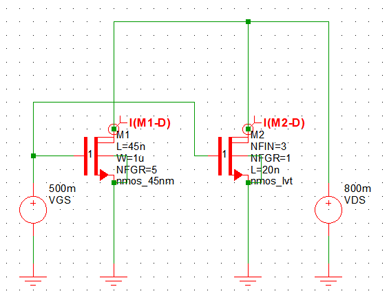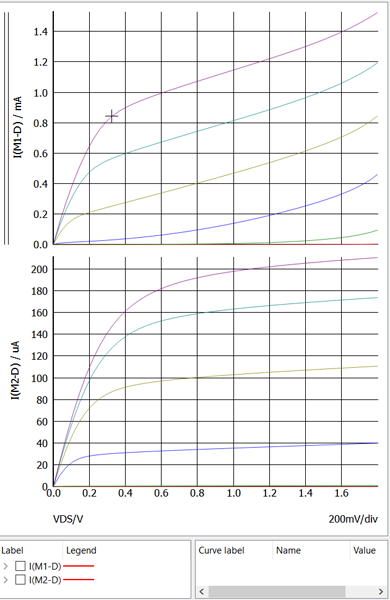### 3.1b. Extract Vt with VDS = 10mV (extrapolating linear part of the ID vs VGS plot)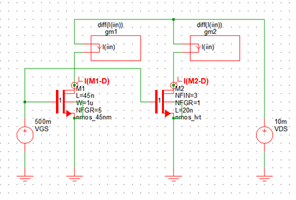Vt at VDS=10 mV is estimated to be 390 mV for the nmos-45nm transistor and 230 mV for the 7 nm FinFET.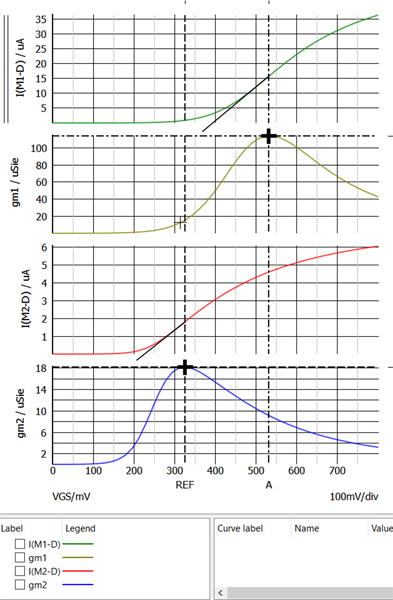### 3.1c. Extract Vt with VDS = 0.8V (extrapolating the linear part of the gm vs. VGS plot).

Vt at VDS=800 mV is estimated to be 270 mV for the nmos-45nm transistor and 230 mV for the 7 nm FinFET.### 3.1d. Compare results from parts (b) and (c ). What's the difference? What might explain this?

The decrease in Vt with increasing VDS is an indication of Drain Induced Barrier Lowering (DIBL) and can be seen in the nmos-45nm transistor and is estimated to be (390 - 270 mV/(10 - 800 mV)= -152 mV/V. My measurements for the 7 nm FinFET are not accurate enough to calculate the a value for DIBL but it is very small as the device shows very little variation in Vt with increasing VDS.

### 3.1e. Plot I‐V characteristics on a semilog scale. What is the subthreshold slope? Is GIDL evident?

The subthreshold slope for the 45 nm and 7 nm nmos transistors are shown below using Id vs VGS. The Subthreshold voltage for the 45 nm NMOS transistor is 100.7 mV/decade and the subthreshold voltage for the 7 nm NMOS transistor is 62.8 mV/decade. Gate Induced Drain Leakage (GIDL) isn't present in either transistor because Id doesn't increase at low VGS voltages.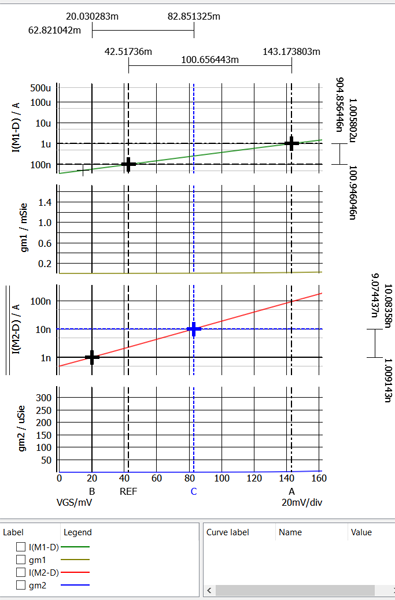### 3.1f. Plot gm/ID vs. VGS (with VDS = 0.8V). How does the value of obtained from plot (i.e. in subthreshold) compare with the subthreshold slope in part (e)?

The subthreshold slope for the 45 nm and 7 nm nmos (bottom) transistors are shown below using gm/ID vs. VGS. The Subthreshold voltage for the 45 nm NMOS transistor is 98.8 mV/decade (top trace) and the subthreshold voltage for the 7 nm NMOS transistor is 62.4 mV/decade (bottom trace). This method shows a slightly smaller subthreshold slope measurement.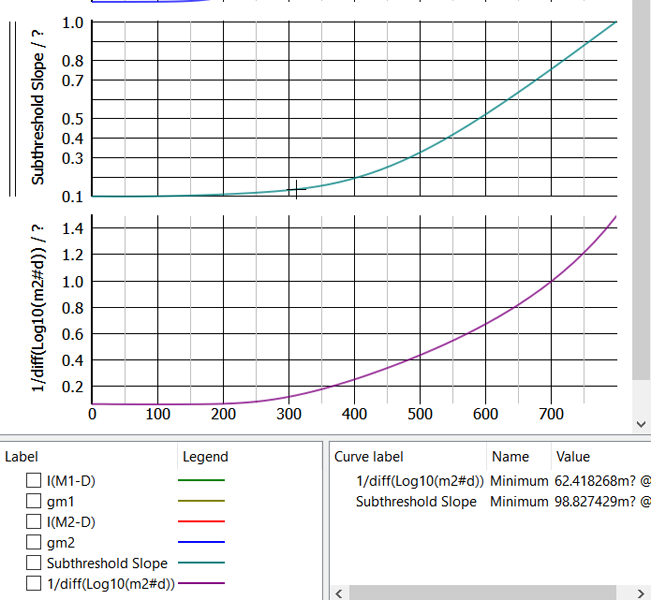### 3.2a. Find intrinsic gain of a transistor with L=45nm. What operating point maximizes the gain? How does this change with L=90nm?

The gate lengths for this problem have been revised to 10 nm and 20 nm because the 7nm nmos_lvt model does not seem to work well with gate lengths greater than 20 nm.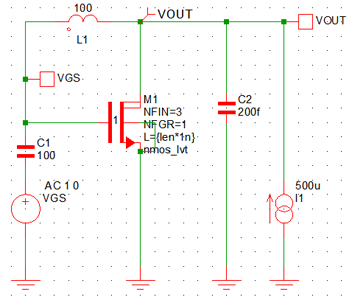The intrinsic gain was extracted from the AC analysis plot below and was found to be 4.9 for the L=20 nm NMOS transistors and the 5.9 for the L=10 nm NMOS transistor at 1 MHz. The model operation is suspect because the 7 nm nmos_lvt model with L=10n provides more gain than the model with L=20n. The longer gate length L=20 should produce more gain [^8] (see pg 38).

### 3.2b. What is the maximum unity‐gain bandwidth achievable when driving a load capacitance of 200 fF with a common‐source transistor while consuming 0.5mW from a 1‐V supply?

The unity gain bandwidth of the L=20 nm NMOS transistor is 186.7 MHz and the L=10 nm NMOS transistor is 183.7 MHz with NFGR=1 and NFIN=3.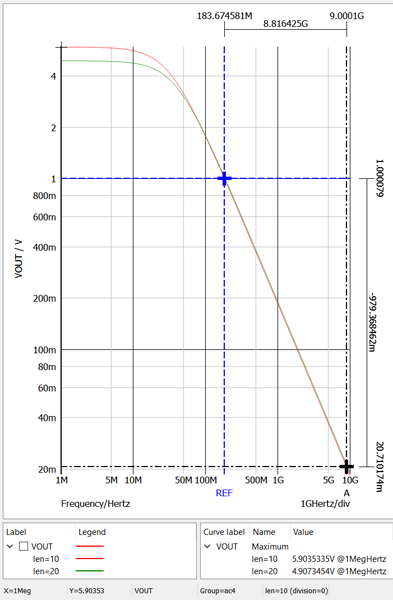### Summary

Summary of the transistor performance is shown in the table below.

Parameter nmos_45nm L=90 nm nmos_45 L=45 nm 7 nm nmos_lvt L=20 nm 7 nm nmos_lvt L=10 nm Units
Vt at VDS=10 mV, Id 390 230 mV
Vt at VDS=800 mV, gm 270 230 mV
Subthreshold at VDS=800 mV, Id 100.7 62.8 mV
Subthreshold at VDS=800 mV, gm 98.8 62.4 mV
n, at VDS=800 mV, Id 1.66 1.05
n at VDS=800 mV, gm 1.68 1.05
Gain at 10 MHz 27.4 5.0 4.9 5.9
Unity Gain Bandwidth 941.7 1307.8 183.7 183.7 MHz

## Problem 4: Repeat homework 1 with two stacked transistors using the same technology as 3 above. Compare the results.

### 4.1a. plot I‐V characteristics

The schematic with the two series 7 nm NMOS transistors and IV curve for Id vs VDS are shown below. The body connection of M1 was connected to its source out of habit, but should have been connected to ground as shown in the schematic below. The results only changed slightly. n -> 1 for the 7 nm nmos-lvt transistors. Lower n reduces or eliminates body effects, improves subthreshold slope, and improves gm/ID (see lecture 2 slide 27).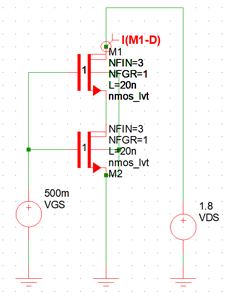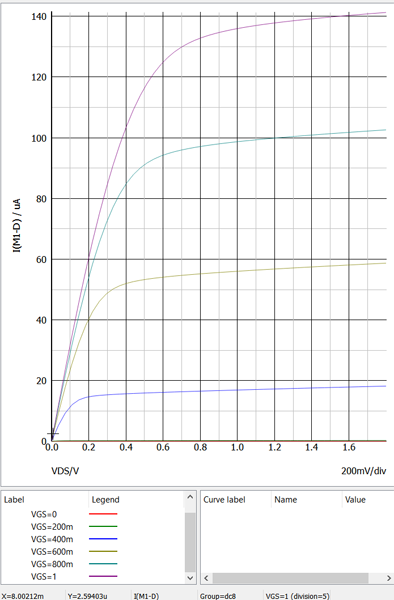### 4.1b. Extract Vt with VDS = 10 mV (extrapolating linear part of the ID vs VGS plot)

Vt at VDS=10 mV is estimated to be 230 mV for the series 7 nm FinFET.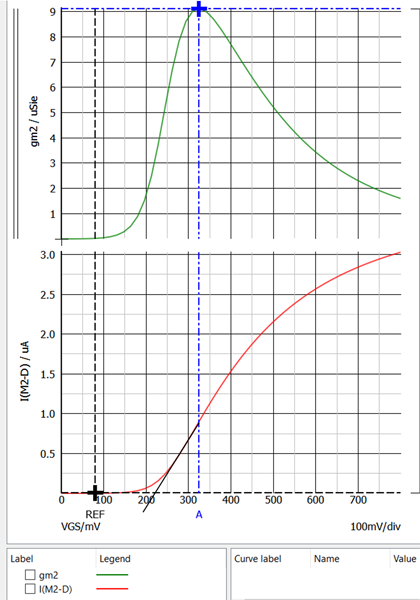### 4.1c. Extract Vt with VDS = 0.8V (extrapolating the linear part of the gm vs. VGS plot).

Vt at VDS=800 mV is estimated to be 230 mV for the series 7 nm FinFET.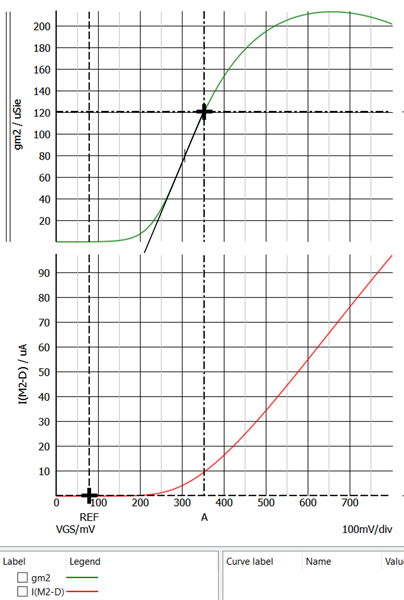### 4.1d. Compare results from parts (b) and (c ). What's the difference? What might explain this?

The 7 nm FinFET shows Very little variation in Vt with increasing VDS so no appreciable DIBL is present in this transistor.

### 4.1e. Plot I‐V characteristics on a semilog scale. What is the subthreshold slope? Is GIDL evident?

The subthreshold slope for the series 7 nm NMOS transistors is shown below using Id vs VGS. The Subthreshold voltage for the series 7 nm NMOS transistor is 62.0 mV/decade. Gate Induced Drain Leakage (GIDL) isn't present in these transistors because Id doesn't increase at low VGS voltages.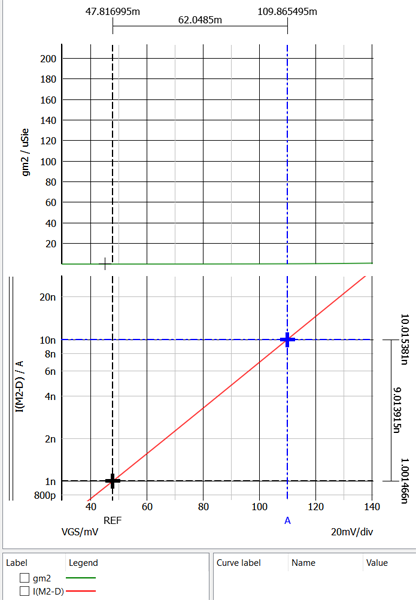### 4.1f. Plot gm/ID vs. VGS (with VDS = 0.8 V). How does the value of obtained from plot (i.e. in subthreshold) compare with the subthreshold slope in part (e)?

The subthreshold slope for series 7 nm nmos transistors is shown below using gm/ID vs. VGS. The Subthreshold voltage for the series 7 nm transistors is 61.7 mV/decade. This method shows a slightly smaller subthreshold slope measurement.### 4.2a. Find intrinsic gain of a transistor with L=45nm. What operating point maximizes the gain? How does this change with L=90nm?

Again, the gate lengths for this problems have been revised to 10 nm and 20 nm because the 7nm nmos_lvt model does not seem to work well with gate lengths greater than 20 nm.

The intrinsic gain was extracted from the AC analysis plot below and was found to be 1.00 for the L=20 nm NMOS transistors at 1.2 kHz, and the 83.4 for the L=10 nm NMOS transistor at 35 kHz.### 4.2b. What is the maximum unity‐gain bandwidth achievable when driving a load capacitance of 200 fF with a common‐source transistor while consuming 0.5mW from a 1‐V supply?

The unity gain bandwidth of the NMOS transistor with L=20 is 52.9 KHz and has some gain peaking at 32 kHz. The unity gain bandwidth of the NMOS transistor with L=10 nm is 109.6 MHz.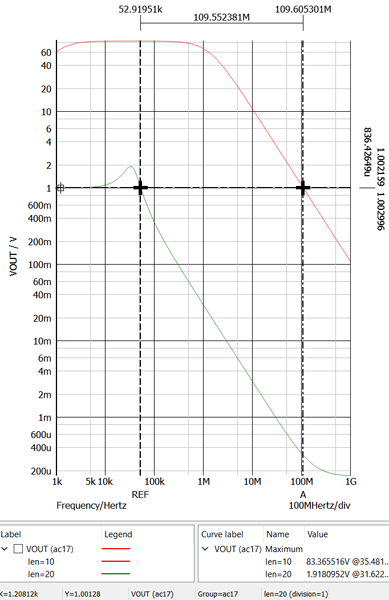### Summary

Summary of the serial transistor performance is shown in the table below.

Parameter 7 nm nmos_lvt L=20 nm 7 nm nmos_lvt L=10 nm Series 7 nm nmos_lvt L=20 nm Series 7 nm nmos_lvt L=10 nm Units
Vt at VDS=10 mV, Id 230 230 mV
Vt at VDS=800 mV, gm 230 230 mV
Subthreshold at VDS=800 mV, Id 62.8 62.0 mV
Subthreshold at VDS=800 mV, gm 52.4 61.7 mV
n, at VDS=800 mV, Id 1.05 1.04
n at VDS=800 mV, gm 1.05 1.04
Gain at 10 MHz/1 kHz 4.9 5.9 1.0 83.4
Unity Gain Bandwidth 183.7 183.7 .0529 109.6 MHz

## Problem 5: Plot ZDS vs. frequency for both transistor in 3 and the stacked-transistor in 4 Compare.

The schematics and plots for ZDS are shown below. The Maximum impedances for each simulation are shown in the table. For the series transistors, ZDS is twice ZDS of the single transistor response. The series transistor produce extra gain but reduce the bandwidth due to doubling the output impedance.

Parameter 7 nm nmos_lvt L=20 nm 7 nm nmos_lvt L=10 nm Series 7 nm nmos_lvt L=20 nm Series 7 nm nmos_lvt L=10 nm Units
ZDS at 1 MHz, VGS = 1 V 1533.4 1385.1 3066.8 2770.2 Ohms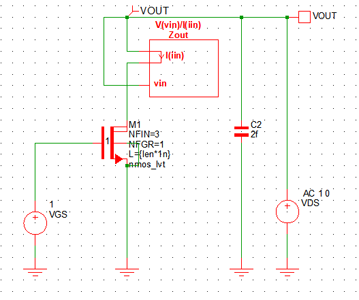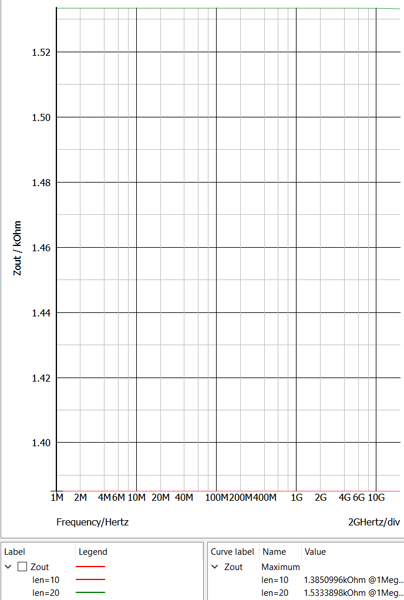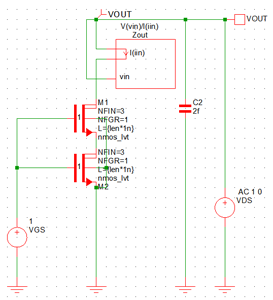## References

The references on the 7 nm transistor models were found on the ASAP7 ASU 7nm PDK website.

: L.T. Clark, V. Vashishtha, L. Shifren, A. Gujja, S. Sinha, B. Cline, C. Ramamurthya, and G. Yeric, ASAP7: A 7-nm FinFET Predictive Process Design Kit, Microelectronics Journal, vol. 53, pp. 105-115, July 2016.

: V. Vashishtha, L. Masand, A. Dosi and L. T. Clark, Systematic Analysis of the Timing and Power Impact of Pure Lines and Cuts Routing for Multiple Patterning, Proc. SPIE DTCO, 2017.

: V. Vashishtha, L. Masand, A. Dosi, and L. T. Clark, Design Technology Co-Optimization of Back End of Line Design Rules for a 7 nm Predictive Process Design Kit, Proc. ISQED, 2017.

: V. Vashishtha, M. Vangala, P. Sharma, and L. T. Clark, Robust 7-nm SRAM Design on a Predictive PDK, Proc. ISCAS, 2017.

: L. T. Clark, V. Vashishtha, D. M. Harris, Samuel Dietrich, and Zunyan Wang, Design Flows and Collateral for the ASAP7 7nm FinFET Predictive Process Design Kit, Proc. MSE, 2017.

: L. T. Clark and V. Vashishtha, , Design with sub-10 nm FinFET Technologies, Presented at CICC, 2017.

: V. Vashishtha, M. Vangala, and L. T. Clark, ASAP7 Predictive Design Kit Development And Cell Design Technology Co-Optimization, Proc. ICCAD, 2017.

: T. C. Carusone, D. A. Johns, and K. W. Martin, Analog Integrated Circuit Design, 2nd Edition. Wiley, 2011.

## Analog IC Design Course Homework 1 SIMetrix Edition

morreale Monday 11 of October, 2021
The Analog IC Design in Nanoscale CMOS short course was held September 7 to October 1, 2021 virtually from the University of Limerick and taught by Professor Tony Chan Carusone. As part of the course, an eight week license to the SIMetrix Technologies SPICE simulator was provided and was used to find solutions to the optional homework problems provided in the class. Here, I show how I used the simulator and the methods presented in the course to find solutions to the problems for homework assignment 1. Homework 1 contains problems 1, 2 and a bonus problem. For more information about the course see Analog IC Design in Nanoscale CMOS Short Course.

For homework 1, we were asked to generate the IV curves for Id vs VDS, Id vs VGS, and gm vs VGS, find the subthreshold slope using Id and gm, and find the unity gain bandwidth for nanoscale transistors using the 45 nm device models with channel lengths of 45 and 90 nm.

Models for 45 nm and 7 nm process transistors were supplied on the course website. The 45 nm models included the low threshold High-speed (vtl) NMOS and PMOS for the typical NMOS and typical PMOS (TT) transistor process variations. The 7 nm models are used in future homework assignments.

## Problem 1: Using 45nm device models

### 1a. plot I‐V characteristics

A schematic was created to plot the IV curves of the transistor using the NMOS_45nm model with length L=45nm, width W=1u, and Number of Fingers NFGR=5. Voltage sources VGS and VDS were added to provide the gate voltage VGS and the drain-source voltage VDS, respectively, to generate the IV-curves.

The model files were added to the SIMetrix model library by dropping them on to the command shell window. The NMOS_45nm model symbol was placed on the on the schematic from the Select Device panel using Place -> From Model Library -> Recently added models.

A current probe was added to measure Id. The probe was placed using Place -> Probe -> Current Probe and then left clicking on the drain pin of the M1. The Simulate -> Choose Analysis set up was configured for a multistep DC sweep of VDS and VGS as shown below.

The IV curve of the NMOS_45nm device is shown for VDS from 0 to 1.8 V, and VGS from 0 to 1 V in steps of 200 mV.

### 1b. Extract Vt with VDS = 10mV (extrapolating linear part of the Id vs VGS plot)

With VDS = 10 mV, the transistor operates in the triode region. The circuit from above was modified by replacing VDS with a battery set to 10 mV and the DC Sweep was set to a single sweep VGS (VGS) from 0 to 800 mV.

The revised schematic is shown below.

An arbitrary probe was added and connected in series with the drain of M1 to measure Id and compute gm using the diff() function. The diff() function returns the derivative of the current I(iin) with respect to the main sweep variable VGS yielding
$$\frac{\partial I_d}{\partial V_{GS}}$$
which is gm. This probe was added using Probe -> Create and Place Arbitrary Probe and it was set up as shown in the figure below. The Axis Type -> Use dedicated plot was selected so that curve appears on its own grid (plot).

gm (top plot) and Id (bottom plot) vs VGS are shown in the plot below.

Using Id vs VGS, Vt was found by projecting the linear region to the VGS axis starting from the peak in the gm vs VGS curve. Vt is estimated to be around 390 mV.

### 1c. Extract Vt with VDS = 0.8V (extrapolating the linear part of the gm vs. VGS plot).

The schematic was modified by setting the battery (VDS) to 800 mV and is shown below.

In the bottom IV curve shown below, Id is linear for VGS > 450 mV indicating that the transistor is operating in the active region. Using gm vs VGS (top plot), Vt was found by projecting the linear region starting from the linear part of the curve to the VGS axis. Vt is estimated to be about 270 mV.

### 1d. Compare results from parts (b) and (c ). What's the difference? What might explain this?

The threshold voltage Vt=390 mV at VDS=10 mV lowers to Vt=270 mV at VDS=800 mV due to the higher drain-source voltage (VDS) and is a result of Drain Induced Barrier Lowering (DIBL).

At VDS=10 mV, the transistor operates in triode mode where
$$I_d=\mu_n C_{ox} (\frac{W}{L})V_{eff}V_{DS}$$
At VDS=800 mV, the transistor operates in the active/saturation region/mode where
$$I_d=\frac{1}{2}\mu_n C_{ox} (\frac{W}{L})V_{eff}^2$$
µn is the nmos carrier mobility, COX is the gate capacitance, W is the channel width, L is the channel Length, Veff is the effective gate voltage (VGS-Vt), and VDS is the drain-source voltages.

### 1e. Plot I‐V characteristics on a semilog scale. What is the subthreshold slope? Is GIDL evident?

The simulation was modified to show gm and Id vs VGS for both VDS voltages of 10 mV and 800 mV. The Choose Analysis panels were configure as shown below.

gm vs VGS is shown in the top plot and Id vs VGS is shown in the bottom plot. For both plots, VDS = 800 mV is the green trace and VDS = 10 mV is the red trace.

Using the Id vs VGS at VDS=800 mV, the subthreshold slope is found to be 100.5 mV/decade. The cursors were dragged to place them a decade a part in the linear part of the current curve to easily determine the subthreshold slope in mV/decade from the voltage difference between the cursors at the top of the plot.

Gate Induced Drain Leakage (GIDL) isn't present in this transistor because Id doesn't increase at low VGS voltages.

### 1f. Plot gm/ID vs. VGS (with VDS = 0.8V). How does the value of obtained from plot (i.e. in subthreshold) compare with the subthreshold slope in part (e)?

gm/ID vs VGS was added to the plot using the Add Curve button and the curve configuration is shown below. m1#d is the Define Curve notation for the drain current Id of transistor M1, and diff() is the derivative function.

Then 1/diff(log10(m1#d)) was added to a grid to plot gm/ID vs. VGS so that the subthreshold slope could be determined using the cursor measurements. The curve is plotted on a new grid by selecting Use new grid.

The new curve is shown below and can be used to determine n using
$$\frac{g_m}{I_d} = \frac{q}{n k T}$$

The subthreshold slope is 99.587 mV/decade using this method (see y=99.587m at the bottom of the figure). n=1.67 was found using
$$Ln(10)\frac{nKT}{q}=subthreshold slope$$
That is
$$n=\frac{99.587 mV}{(2.3kT/q)}$$

## Problems 2: Also using 45nm device models

### 2a. Find intrinsic gain of a transistor with L=45nm. What operating point maximizes the gain? How does this change with L=90nm?

The calculations for the intrinsic gain |Ai| and rds are shown below.
$$\frac {1}{r_{ds}}=g_{ds}=\frac{\partial I_d}{\partial V_{DS}}=\lambda I_d$$
$$|A_i|=g_m r_{ds}$$
The intrinsic gain was extracted from the AC analysis plot and was found to be 5.03 for the L=45 nm NMOS transistors and the 27.38 for the L=90 nm NMOS transistor at 10 MHz. Notice that the transistor with the shorter length (L=45 nm) has lower gain and more bandwidth, and the transistor with the longer channel length (L=90 nm) has more gain and lower bandwidth (see Carusone et. al. pg. 38).

### 2b. What is the maximum unity‐gain bandwidth achievable when driving a load capacitance of 200 fF with a common‐source transistor while consuming 0.5mW from a 1‐V supply?

The schematic shows the nmos_45nm transistor with a bias circuit of L1 and C2 driven by a 1 V AC source. C1 is the 200 fF load and I1 is the 500 µA supply current.

The Choose Analysis configuration is shown below. The transistor length len is generated from in the Define List containing 45 and 90. These values are multiplied by 1n in the model definition for L={len*1n} (see M1 in the schematic).

The 3 dB bandwidth of the 90 nm NMOS transistor is 63.9 MHz and the 45 nm NMOS transistor is 453.3 MHz.

The unity gain bandwidth of the 90 nm NMOS transistor is 941.7 MHz and the 45 nm NMOS transistor is 1307.8 MHz.

### You may repeat these exercises for other model files available at: Model files

For example, the exercises can be repeated for SS/FF corners, PMOS devices, etc.

The exercise was recomputed for the pmos_45nm transistors since only the typical models for the 45 nm devices were available on the course website. The schematic and IV curve for the PMOS_45nm transistor is shown below. The parameters for the nmos-45nm transistor were used again for the pmos_45nm transistor (L=45n, W=1u, NFGR=5).

The Choose Analysis DC VGS sweep of the PMOS_45nm transistor setup is shown in the figure below.

Vt is estimated to be 400 mV at VDS=10mV using Id.

Vt is estimated to be 215 mV at VDS=800 mV using gm. The lower Vt indicated DIBL is present in this device.

The subthreshold slope is 105.7 mV/decade using Id at VDS=800 mV. n=1.78 was calculated using this subthreshold slope.

Added the curve 1/diff(log10(m2#s)) to a new plot (top plot) to determine the subthreshold slope using
$$\frac{1}{\frac {\partial Log10(I_s)}{\partial V_{GS}}}$$
as shown in the Define Curve configuration shown below. In this PMOS transistor m2#d (Id) is negative so m2#s is the source current and is used to keep the plot value positive so that log scales can be used.

Using the cursor on the green curve in the top plot, the subthreshold slope is 96.2752 mV/decade. This subthreshold slope yields n=1.62. Id does not increase at low VGS so GIBL isn't detected in this device.

From the AC plot below the intrinsic gain for the 45 nm PMOS transistors is 2.9 and the gain for the 90 nm NMOS transistor is 5.8 at 10 MHz. The unity gain bandwidth of the 90 nm PMOS transistor is 225.15 MHz and the 45 nm PMOS transistor is 563.69 MHz.

### Summary

Summary of the transistor performance is shown in the table below.

 Parameter nmos_45nm L=90 nm nmos_45 L=45 nm pmos_45nm L=90 nm pmos_45nm L=45 nm Units Vt at VDS=10mv, Id 390 400 mV Vt at VDS=800mv, gm 270 215 mV Subthreshold at VDS=800mv, Id 100.5 105.7 mV Subthreshold at VDS=800mv, gm 99.587 96.3 mV n, at VDS=800mv, Id 1.69 1.78 n at VDS=800mv, gm 1.67 1.62 Gain at 10 MHz 27.4 5.0 5.8 2.9 Unity Gain Bandwidth 941.7 1307.8 225.2 563.7 MHz

## Acknowledgements

I really enjoyed this course. I appreciate Professor Carusone for teaching the course, Hooman Reyhani for his efforts organizing the course, and John Warner for providing the licenses for the SIMetrix Technologies simulator.

## MRS Material Science for Quantum Computing Webinar References

morreale Saturday 17 of July, 2021

The Materials Research Society (MRS) held the webinar Material Science for Quantum Computing recently, and it is available on demand now. The moderator was Bob Braughler (MRS) and the introduction was given by Vincenzo Lordi from Lawrence Livermore National Laboratories (LLNL). The talk topics and speakers included:

• Atomic-precision advanced manufacturing for Si quantum computing by Ezra Bussmann.
• Novel Characterization of the Quantum States of Single-Spin Qubits (revised to Novel Characterization of Donor Qubits in Silicon) by Benoit Voisin.
• Characterization and Control of Diamond Surfaces for Qubits and Novel Materials probes by Shimon Kolkowitz.

All the talks were interesting and informative. Slides for the talks were not available but the following references were provided.

morreale Friday 09 of July, 2021

The Analog IC Design in Nanoscale CMOS short course is scheduled for September 7 to October 1, 2021 virtually from the University of Limerick. The short course consists of eight 1.5 hour lectures followed by a half hour question and answer session. The course is taught by Professor Tony Chan Carusone.

## Course Program

• Lecture 1 - CMOS Device Scaling & Modeling 7th Sept. 2021 Device scaling, short-channel length effects, analog design on bulk CMOS technologies below 30nm.
• Lecture 2 - Advanced CMOS Technologies: SOI & FinFET 10th Sept. 2021 Impact on transistor model parameters, analog FOM & layout strategies. Reliability effects.
• Lecture 3 - Amplifier Design in Nanoscale CMOS 14th Sept. 2021 Design of current mirrors & amplifier circuits. Suitable OTA topologies for low supply-voltage.
• Lecture 4 - References, Regulators & Power Integrity 17th Sept. 2021 Diff. reference circuits & voltage regulation. Power distribution in ICs. Power integrity analysis.
• Lecture 5 - Nanoscale CMOS Clocking 21st Sept. 2021 Jitter sources, amplification & power-supply-induced jitter. CMOS buffering & clock distribution.
• Lecture 6 - Dynamic Comparators & Amplifiers 24th Sept. 2021 Applications of dynamic amplifiers (as integrators) in high-speed receivers. Dynamic comparators.
• Lecture 7 - ADC-Based Receivers in Nanoscale CMOS 28th Sept. 2021 High-speed ADCs: folding-flash, binary search & CT pipelined. Combatting mismatch & applications.
• Lecture 8 - Real-Life Cautionary Tales 1st Oct. 2021 Common design & layout errors, integrating large designs, power & clock routing, non-working chips.

## References

The following references provides some background for the course.

• Textbook chapter 1 for lectures 1 and 2
• Textbook chapter 7.4 for lecture 4
• IEEE articles shown below

## Text Book## CNF Technology & Characterization at the Nanoscale Virtual Short Course

morreale Sunday 14 of February, 2021

The CNF Technology & Characterization at the Nanoscale virtual short course was held online January 27 to 29, 2021. The course was excellent and gives an overview of nanofabrication techniques various applications using various method and tools. The course also provided an overview of the fabrication capabilities at the CNF. The CNF staff has a deep background in nanofabrication and recipe development and is a key benefit of working at the CNF.

## Day 1

• Welcome & Introduction to the CNF
• Overview of Microfluidics
• Introduction to photolithography
• Lab Demonstration 1: Microfluidic Device Fabrication - Photolithography
• Introduction to the Fabrication of Microfluidics
• Introduction to Etching and Pattern Transfer
• Lab Demonstration 2: Microfluidic Device Fabrication - Deep Reactive Ion Etching (DRIE), Profilometry, Surface modification (MVD), PDMS Mixer & assembly

## Day 2

• Introduction to MEMS
• Thin Films, Thermal Oxidation, Chemical Vapor Deposition, and Thin Film Characterization
• Lab Demonstration 3: Chemical Vapor Deposition (LPCVD, PECVD)
• Projection Photolithography
• Lab Demonstration 4: Projection Photolithography (Steppers)
• Reactive Ion Etching, Wet Etching & Release
• Lab Demonstration 5: Plasma Etching
• Scanning Electron Microscopy
• Lab Demonstration 6: Scanning Electron Microscopy (SEM)

## Day 3

• Introduction to Fabricating Nano-electrodes
• Electron Beam Lithography, Nano Imprint Lithography
• Lab Demonstration 7: Electron Beam Lithography
• Physical Vapor Deposition, Pattern Transfer - Lift Off
• Lab Demonstration 8: Evaporation/ Sputter
• Atomic Force Microscopy
• 2D-Materials: Graphene and Beyond
• Lab Demonstrations 9: Atomic Force Microscopy (AFM), (ALD), (ALE)
• Process Integration Considerations

## The Nanoscale

A nanometer is wicked small. For comparison, the ratio of the moon's orbit around the Earth (~4 * 10⁸ meters) to a ruler (about 15 inches) is 10⁹. The ratio of the ruler to the radius of a strand of DNA (1 nm) is also 10⁹. This nanoscale realm makes fabricating devices and structures challenging. Developing a device at the nanoscale involves a cyclical process as shown in figure below.### Process DRC and Monitoring

The short course concentrated mostly on fabrication as it is considered the most difficult aspect of entire process. During the layout phase it's important to preform design rule checks (DRC) to ensure that fabrication facility can produce the device. Process control monitors (PCM) are included in the layout to help verify the performance of the device. Standard test structures are included in the layout for this purpose and are commonly used in commercial wafer fabs, and are recommend in at user fabs. These structures include: Van der Pauw structures for measuring sheet resistance, contact chains used to identify shorts and opens, Kelvin structures to measure contact resistance, and grating to monitor critical dimensions.

### Process Flow

Fabrication involves integrating discrete steps together to create a process flow to make a device. The process flow depends on the materials used, the geometry of the device structures, and interconnects. Creating a process flow requires knowledge of many disciplines and can be complicated. It involves selecting a photolithography process, etch, and deposition method that is compatible with the materials selected.

### Photolithography

Wafers can be patterned using contact or projection photolithography. With contact photolithography, a mask is placed in contact with a wafer coated with photoresist and exposed with UV light. The whole wafer is exposed at once. With projection photolithography, a pattern is projected onto a section of the wafer coated with photoresist and is exposed. The wafer is moved and the exposure is repeated until all areas of the wafer have been exposed. This is known as step and repeat photolithography.

The photoresist is selected depending on the type of photolithography used, the type of etching to be used, the depth of etch, the materials to be etched, and the materials to be deposited. Both positive and negative resistors are available too.

### Deposition

Dielectric thin films can be deposited on a substrate using many techniques including Chemical Vapor Deposition (CVD), Low Pressure CVD (LPCVD), Plasma-Enhanced CVD (PECVD), Atomic Layer Deposition (ALD). Each deposition method produces a thin film with unique properties such as density, quality, uniformity, and step coverage with variations in deposition rate, deposition temperature, and batch processing rate.

Metal thin films are deposited using Physical Vapor Deposition (PVD) that involve thermal and electron beam evaporation, sputtering, reactive evaporation, reactive sputtering, and co-evaporation.

### Etch

Once a wafer is patterned, it can be etched using wet etching (also known as chemical etching), plasma etching, ion etching, and Reactive Ion Etching {RIE}. Etching removes thin films, the substrate, or both. Wet etching uses chemicals like Potassium Hydroxide (KOH) to remove silicon anisotropically, or Hydrofluoric (HF) acid to remove Silicon Dioxide (SiO₂) isotropically, for example. Plasma etching uses ionized gas to remove materials through physical bombardment of the surface. Ion etching use an ion beam to remove materials by accelerating ion at the surface and is also known as ion milling. RIE introduces reactive chemicals that react with the surface materials into the plasma.

## CNF Certificate of Completion

A few days after the course, I received my certificate of completion.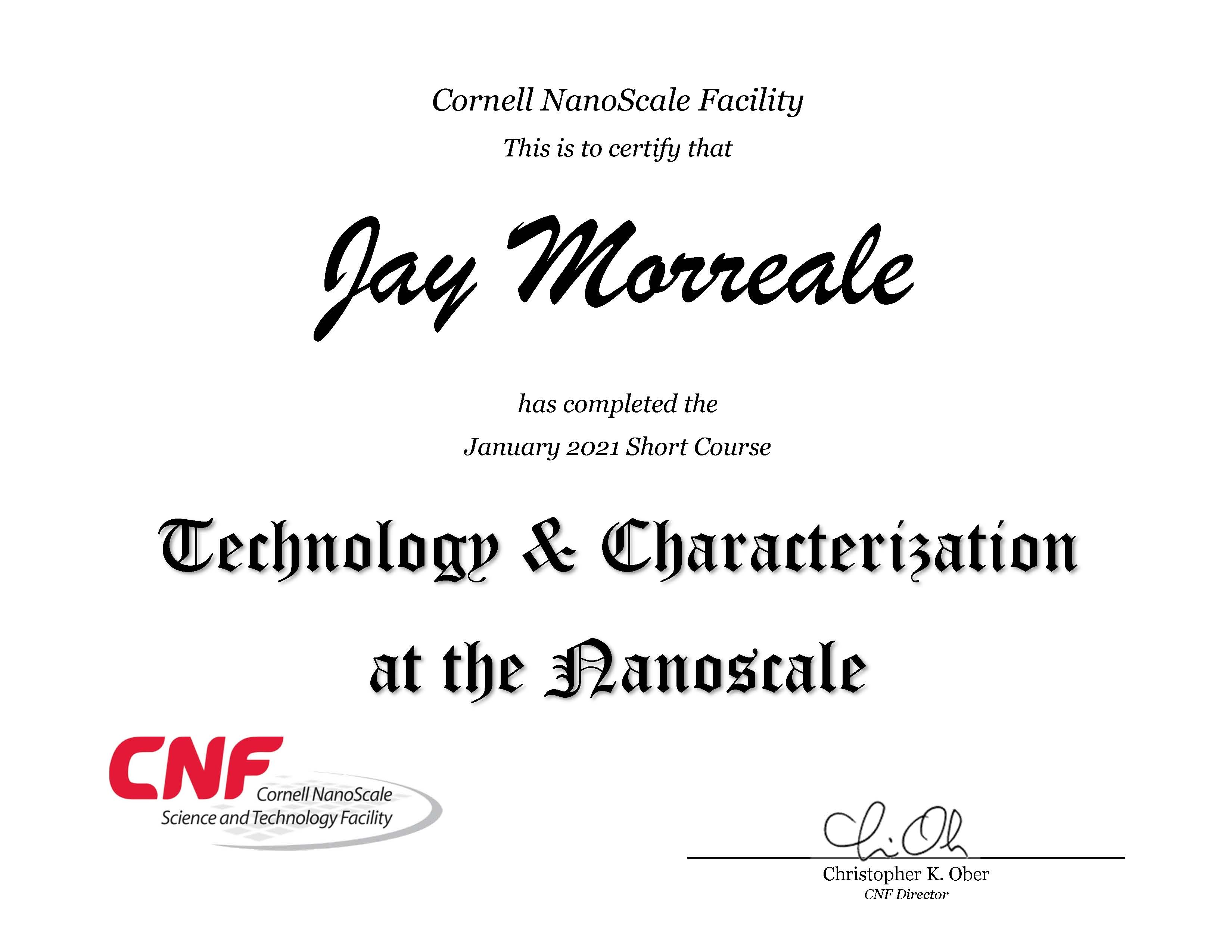## References

• CNF Technology & Characterization at the Nanoscale workbook, January 27-29, 2021.
• Process Integration by Christopher Alpha, January 29, 2021.

## Cornell Technology and Characterization at the Nanoscale Short Couse Jan 27-29, 2021

morreale Friday 08 of January, 2021
The CNF is running a virual short course on Technology & Characterization at the Nanoscale on January 27-29, 2021. The course topics include:

• Wednesday, Day 1: Introduction & Microfluidic Systems (11:00am - 3:00pm EST)
• Thursday, Day 2: MEMS Cantilever Fabrication (11:00am - 3:00pm EST)
• Friday, Day 3: Nanoelectrode Fabrication (11:00am - 3:00pm EST)
The course is open the the general scientific community and costs \$60 to register.